##### The Japanese Bonsai specialist
Direct order Contact Help / Services Newsletter# Pinus pentaphylla ref :220802341260

Pinus pentaphylla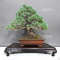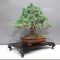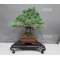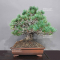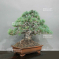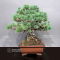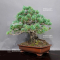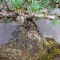ref. : 12016Product unavailable

###### Description

Exceptional bonsaï: a treasure for collectors.
This remarkable bonsaï captures the very essence of bonsai art and stands as a true gem for collectors.

The tree, with an imposing height of 345 mm, displays a majestic span of branches extending over 380 mm.

Its pottery, a non-glazed Japanese artisanal creation, attests to unparalleled quality, measuring 260*180*70 mm.

The moyogi style in which it is designed enhances its natural and balanced charm. The pine branches are gracefully arranged, evoking a perfect harmony, without the slightest trace of visible wires or scars from past cutting.

The trunk diameter, measures between 90 and 100 mm. I

ts nebari, magnificently spread over 130-150 mm, adds a striking touch of elegance. This variety of pinus, specifically chosen for its short needle length and vivid green hue slightly tinged with blue, is an exceptional selection from seedlings.

The bark, shaped by the passage of time over more than 50 years, bears witness to the acquired maturity and wisdom.

Originating from the nurseries of Master Katoh Katsuhiro, this tree is truly extraordinary, a rarity that elicits admiration. Its features are elevated by the perfectly conical trunk, a characteristic that bestows upon this bonsai a unique charm.

In February 2020, Master Tomoya Nishikawa meticulously reworked this tree to further enhance its beauty.

His expertise elevated each detail to achieve an ultimate aesthetic balance. In Japan, it is rare to find a five-needle pine of such grace and size.

The mere contemplation of this masterpiece evokes deep emotion in every observer.

This photograph was taken in August 2023. Please note that the display stand is not included.

#bonsai 6.6 #this 6.4 #pinus 3.5 #from 3.4 #that 3.4 #over 3.4 #tree 3.4 #masterpieces 3.2 #pentaphylla 3.1 #exceptional 3.1

Formule
(( ROUND((CHAR_LENGTH(b.article_nom)-CHAR_LENGTH(REPLACE(b.article_nom, 'this', '')))/LENGTH('this')) + ROUND((CHAR_LENGTH(b.article_description)-CHAR_LENGTH(REPLACE(b.article_description, 'this', '')))/LENGTH('this')) ) * 6.4) + (( ROUND((CHAR_LENGTH(b.article_nom)-CHAR_LENGTH(REPLACE(b.article_nom, 'bonsai', '')))/LENGTH('bonsai')) + ROUND((CHAR_LENGTH(b.article_description)-CHAR_LENGTH(REPLACE(b.article_description, 'bonsai', '')))/LENGTH('bonsai')) ) * 4.6) + (( ROUND((CHAR_LENGTH(b.article_nom)-CHAR_LENGTH(REPLACE(b.article_nom, 'pinus', '')))/LENGTH('pinus')) + ROUND((CHAR_LENGTH(b.article_description)-CHAR_LENGTH(REPLACE(b.article_description, 'pinus', '')))/LENGTH('pinus')) ) * 3.5) + (( ROUND((CHAR_LENGTH(b.article_nom)-CHAR_LENGTH(REPLACE(b.article_nom, 'tree', '')))/LENGTH('tree')) + ROUND((CHAR_LENGTH(b.article_description)-CHAR_LENGTH(REPLACE(b.article_description, 'tree', '')))/LENGTH('tree')) ) * 3.4) + (( ROUND((CHAR_LENGTH(b.article_nom)-CHAR_LENGTH(REPLACE(b.article_nom, 'from', '')))/LENGTH('from')) + ROUND((CHAR_LENGTH(b.article_description)-CHAR_LENGTH(REPLACE(b.article_description, 'from', '')))/LENGTH('from')) ) * 3.4) + (( ROUND((CHAR_LENGTH(b.article_nom)-CHAR_LENGTH(REPLACE(b.article_nom, 'over', '')))/LENGTH('over')) + ROUND((CHAR_LENGTH(b.article_description)-CHAR_LENGTH(REPLACE(b.article_description, 'over', '')))/LENGTH('over')) ) * 3.4) + (( ROUND((CHAR_LENGTH(b.article_nom)-CHAR_LENGTH(REPLACE(b.article_nom, 'that', '')))/LENGTH('that')) + ROUND((CHAR_LENGTH(b.article_description)-CHAR_LENGTH(REPLACE(b.article_description, 'that', '')))/LENGTH('that')) ) * 3.4) + (( ROUND((CHAR_LENGTH(b.article_nom)-CHAR_LENGTH(REPLACE(b.article_nom, 'pentaphylla', '')))/LENGTH('pentaphylla')) + ROUND((CHAR_LENGTH(b.article_description)-CHAR_LENGTH(REPLACE(b.article_description, 'pentaphylla', '')))/LENGTH('pentaphylla')) ) * 3.1) + (( ROUND((CHAR_LENGTH(b.article_nom)-CHAR_LENGTH(REPLACE(b.article_nom, 'exceptional', '')))/LENGTH('exceptional')) + ROUND((CHAR_LENGTH(b.article_description)-CHAR_LENGTH(REPLACE(b.article_description, 'exceptional', '')))/LENGTH('exceptional')) ) * 3.1) + (( ROUND((CHAR_LENGTH(b.article_nom)-CHAR_LENGTH(REPLACE(b.article_nom, 'collectors', '')))/LENGTH('collectors')) + ROUND((CHAR_LENGTH(b.article_description)-CHAR_LENGTH(REPLACE(b.article_description, 'collectors', '')))/LENGTH('collectors')) ) * 3)

## Secure payment## Delivery

Our logistic partners :04 74 55 23 48
Pépinière MAILLOT-BONSAÏ
Le Bois Frazy
01990 RELEVANT - FRANCE
on appointment